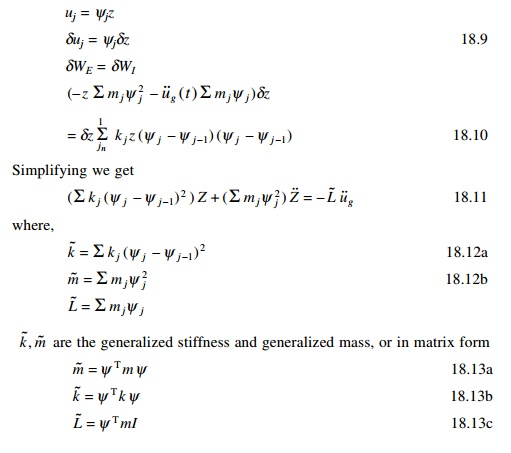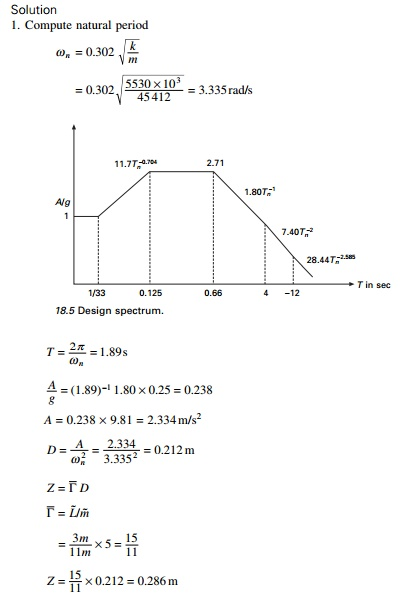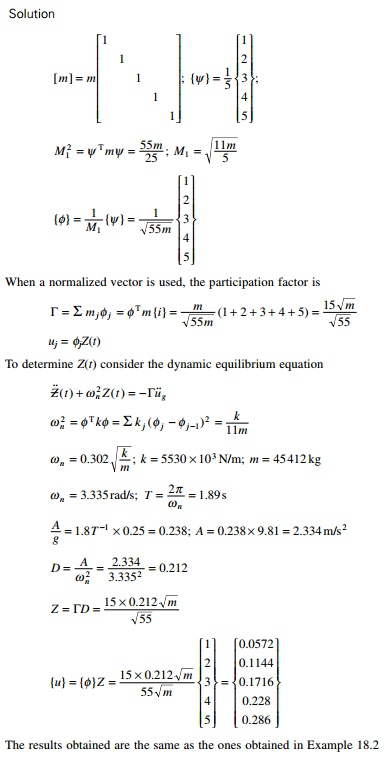Home | | Structural Dynamics and Earthquake Engineering | Lumped mass system: shear building

# Lumped mass system: shear building

The masses of N stories are lumped at these levels with mj denoting the mass at the jth floor.

Lumped mass system: shear building

Consider the shear building shown in Fig. 18.1 subjected to earthquake excitations. The masses of N stories are lumped at these levels with mj denoting the mass at the jth floor. The system has n degrees of freedom u1, u2 ŌĆ” un. Assume there is no damping.

We assume floor displacement relative to the ground can be expressed asWhere z and Žł are the displacement of the roof and shape function respectively.

Hence total displacement1  Equation of motion

Shear Vj in the jth storey can be given as

Vj = kj (storey drift)

= kj Ōłåj

kj(uj ŌĆō ujŌĆō1)kj, the storey stiffness, is the sum of all lateral stiffness of all columns to that storeywhere EI is the flexural rigidity of the column and h is the storey height. At each time instant the system is in equilibrium under the action of

internal storey shear and inertia force and using DŌĆÖAlembertŌĆÖs principle we get

Using the principle of virtual displacement to formulate equilibrium equation, the external virtual work can be written asThe internal virtual work is due to storey shear Vj(t) acting through storey driftsNow we can express displacement in terms of generalized coordinates aswhere Ōłåj, the stories drift given in terms of storey displacements (see Fig. 18.2), {I} is a vector with all elements equal to unity. The equations denoted above are not only applicable to shear building but also to all structures.

Consider a three storey frame shown in Fig. 18.3. It can be proved,2  Response analysiswhere h is the height of the floor above base. In particular the shear and over-turning moment at the base areExample 18.1

The uniform five storey shear frame with rigid beams shown in Fig. 18.4 is subjected to ground acceleration. All the floor masses are m and all stories have same height and stiffness k. Assume the displacement to increase linearly with height above base; formulate the equation of motion for the system and determine natural frequency.Example 18.2

Determine the peak displacement, storey shears, floor overturning moment for the above frame with m = 45 412 kg, k = 5530 kN/m; h = 3.657 m due to ground motion characterized by design spectrum scaled to peak ground acceleration of 0.25g. The spectrum is given in Fig. 18.5.Example 18.3

Solve Example 18.1 using a normalized vector Žå instead of Žł.

The results obtained are the same as the ones obtained in Example 18.2Study Material, Lecturing Notes, Assignment, Reference, Wiki description explanation, brief detail
Civil : Structural dynamics of earthquake engineering : Lumped mass system: shear building |